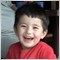# Why is my indicator showing 0 all the time?701

Hi everybody,

I'm trying to make a new indicator to work as a kind of proof of concept test for an idea that i had, but i can't get it to work to test my idea. I have some experience with the language, but I mainly code EA's, so coding indicators is pretty new to me.

here is the code I am trying to use in the indicator right now:

any help is appreciated and if you can explain why something needs to be a certain way that would be great as well because it would help to know for future reference.

```#property copyright "Copyright 2010, MetaQuotes Software Corp."
#property version   "1.00"
#property indicator_separate_window
#property indicator_minimum -100
#property indicator_maximum 100
#property indicator_buffers 1
#property indicator_plots   1
//--- plot Susceptibility
#property indicator_label1  "Susceptibility"
#property indicator_type1   DRAW_LINE
#property indicator_color1  DarkGreen
#property indicator_style1  STYLE_SOLID
#property indicator_width1  1
//--- indicator buffers
double         SusceptibilityBuffer[];
//+------------------------------------------------------------------+
//| Custom indicator initialization function                         |
//+------------------------------------------------------------------+
int OnInit()
{
//--- indicator buffers mapping
SetIndexBuffer(0,SusceptibilityBuffer,INDICATOR_DATA);
//--- indicator accuracy
IndicatorSetInteger(INDICATOR_DIGITS,2);
//--- start drawing of line
PlotIndexSetInteger(0,PLOT_DRAW_BEGIN,3);
//--- set line name
PlotIndexSetString(0,PLOT_LABEL,"Susceptibility to Change");
//--- short name
IndicatorSetString(INDICATOR_SHORTNAME,"Susceptibility");
return(0);
}
//+------------------------------------------------------------------+
//| Custom indicator iteration function                              |
//+------------------------------------------------------------------+
int OnCalculate(const int rates_total,
const int prev_calculated,
const datetime& time[],
const double& open[],
const double& high[],
const double& low[],
const double& close[],
const long& tick_volume[],
const long& volume[],
{
if(rates_total<1)
return(0);
int i;
double o,h,l,c;
for(i=prev_calculated-1;i<rates_total;i++)
{
o = open[i];
h = high[i];
l = low[i];
c = close[i];
SusceptibilityBuffer[i] = (h-l)/MathAbs(o-c);
}
//--- return value of prev_calculated for next call
return(rates_total);
}
//+------------------------------------------------------------------+

```1053

PipSlayer42:

Hi everybody,

I'm trying to make a new indicator to work as a kind of proof of concept test for an idea that i had, but i can't get it to work to test my idea. I have some experience with the language, but I mainly code EA's, so coding indicators is pretty new to me.

here is the code I am trying to use in the indicator right now:

any help is appreciated and if you can explain why something needs to be a certain way that would be great as well because it would help to know for future reference.

PipSlayer42,

prev_calculated start in 0. So your for statement, the i variable starts in -1, causing "Array out of range" error.

Try to include the line:

for(i=prev_calculated-1;i<rates_total;i++)
{

if (i < 0) continue;
o = open[i];
h = high[i];
l = low[i];
c = close[i];
SusceptibilityBuffer[i] = (h-l)/MathAbs(o-c);
}

Also, if, by chance, open[i] equals to close[i], in the calculation of SusceptibilityBuffer[i] you are dividing by 0, causing error.

Regards,

Jin701

Jin:

PipSlayer42,

prev_calculated start in 0. So your for statement, the i variable starts in -1, causing "Array out of range" error.

Try to include the line:

for(i=prev_calculated-1;i<rates_total;i++)
{

if (i < 0) continue;
o = open[i];
h = high[i];
l = low[i];
c = close[i];
SusceptibilityBuffer[i] = (h-l)/MathAbs(o-c);
}

Also, if, by chance, open[i] equals to close[i], in the calculation of SusceptibilityBuffer[i] you are dividing by 0, causing error.

Regards,

Jin

i tried adding in the extra if statement, but it didn't really change anything. On the h1 chart, the indicator only shows 0 for every value, but on the h4 chart it shows 1.36 and huge random values for no reason at random points. Thanks for trying to help though, and i have thought about that last issue. i'll probably add in another if statement to fix it, but the constant 0's are my main concern. thanks again though701

i got it working. i added in this if statement after equating o, h, l, and c

if(o-c == 0.00000)

o+=0.00001;

to solve the issue of if the open minus close was ever equal to zero and it all just started working. Now i know that i still need some refinement, but thank you for your help